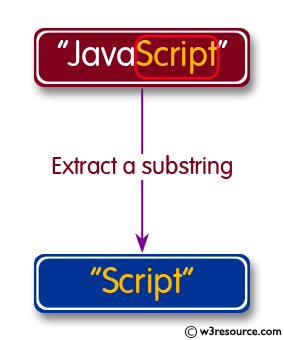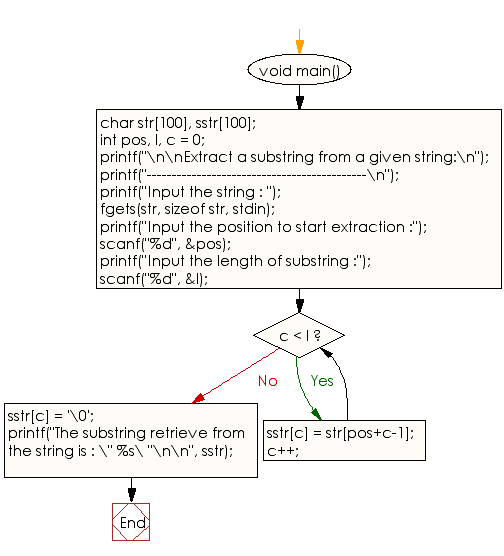﻿ C exercises: Extract a substring from a given string - w3resource# C Programming: Extract a substring from a given string

## C String: Exercise-13 with Solution

Write a program in C to extract a substring from a given string.Sample Solution:

C Code:

``````#include <stdio.h>
void main()
{
char str, sstr;
int pos, l, c = 0;

printf("\n\nExtract a substring from a given string:\n");
printf("--------------------------------------------\n");

printf("Input the string : ");
fgets(str, sizeof str, stdin);

printf("Input the position to start extraction :");
scanf("%d", &pos);

printf("Input the length of substring :");
scanf("%d", &l);

while (c < l)
{
sstr[c] = str[pos+c-1];
c++;
}
sstr[c] = '\0';

printf("The substring retrieve from the string is : \" %s\ "\n\n", sstr);

}
```
```

Sample Output:

```Extract a substring from a given string:
--------------------------------------------
Input the string : This is test string
Input the position to start extraction :9
Input the length of substring :4
The substring retrieve from the string is : " test "
```

Flowchart :C Programming Code Editor:

Improve this sample solution and post your code through Disqus.

What is the difficulty level of this exercise?

﻿